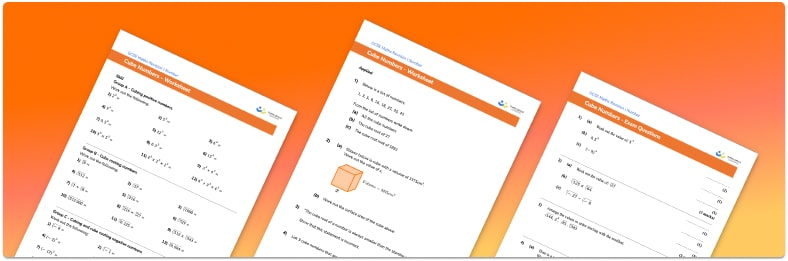# Cube Numbers Worksheet• Section 1 of the cube numbers worksheet contains 20+ skills-based cube numbers questions, in 3 groups to support differentiation
• Section 2 contains 3 applied cube numbers questions with a mix of word problems and deeper problem solving questions
• Section 3 contains 3 foundation and higher level GCSE exam style cube numbers questions
• Cube numbers answers and a mark scheme for all cube numbers questions are provided
• Questions follow variation theory with plenty of opportunities for students to work independently at their own level
• All questions created by fully qualified expert secondary maths teachers, based on the national curriculum
• Suitable for GCSE maths revision for AQA, OCR and Edexcel exam boards

• This field is for validation purposes and should be left unchanged.

You can unsubscribe at any time (each email we send will contain an easy way to unsubscribe). To find out more about how we use your data, see our privacy policy.

### Cube numbers at a glance

Cube numbers are the result when a number is multiplied by itself twice. For example, 5*5*5=125 so 125 is a cube number. The first five cube numbers are 1, 8, 27, 64 and 125.

The cube root of a number is the number that you cube to get that number, so the cube root of 125 is 5. There is no easy way to find the cube root of a number, it is best to learn the first 5 cube numbers.

The cube of a negative number is negative e.g. -2 * -2 * -2 = -8 and so we can cube root negative numbers whereas we cannot square root negative numbers.

Looking forward, students can then progress to additional number worksheets, for example a percentage worksheet or a rounding worksheet.For more teaching and learning support on Number our GCSE maths lessons provide step by step support for all GCSE maths concepts.

## Do you have KS4 students who need more focused attention to succeed at GCSE?There will be students in your class who require individual attention to help them succeed in their maths GCSEs. In a class of 30, it’s not always easy to provide.

Help your students feel confident with exam-style questions and the strategies they’ll need to answer them correctly with our dedicated GCSE maths revision programme.

Lessons are selected to provide support where each student needs it most, and specially-trained GCSE maths tutors adapt the pitch and pace of each lesson. This ensures a personalised revision programme that raises grades and boosts confidence.

Find out more